# Astronomy 12 - Spring 1999 (S.T. Myers)

## The Tully-Fisher and Faber-Jackson Relations

There are 3 "observable" physical properties of galaxies, R, L, and M, as there are in stars. These are given by the relations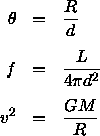The distance d enters into the small-angle relation and the flux-luminosity relation. We can eliminate this between the two using the surface brightness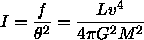which is independent of the distance, as we discussed in Lecture 3. We can rearrange this to find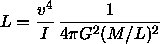where we have introduced the mass-to-light ratio M/L. If we can assume that for a given class of galaxies that the central surface brightness I (in Lsun/pc^2) and the mass-to-light ratio M/L are constant, then we find the relation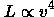which is the basis of some of the most useful distance indicators in cosmology! For instance, if we are observing spiral galaxies, the appropriate velocity to use is the maximum velocity v_max in the rotation curve which can be determined from H I spectra. Then, we havewhich is known as the Tully-Fisher relation. On the other hand, if we are concerned with elliptical galaxies, the appropriate velocity is the central velocity dispersion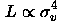which is known as the Faber-Jackson relation. Both of these relations were discovered empirically and are justified through the arguments given above. The proportionality must be calibrated using galaxies with known distances, then the relations can be used to find the luminosity, and thus the distance, given an observation of the apparent magnitude and velocity width. For example, a typical relation for ellipticals is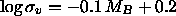where MB is the absolute galaxy magnitude (to some isophotal level) in the B band.

Note that it is still under debate whether these relations are universal, that is, whether I and M/L are dependent only on the galaxy type, and do not depend on other things such as location and environment.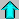Astro12 Index ---Astro12 Home

smyers@nrao.edu Steven T. Myers